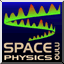#Drift mechanisms

SpaceWeb@SkyNet.oulu.fi - last update: 2 January 1997, 1400 UT (RR)
In the presence of only uniform magnetic field B, the motion of a charged particle can be described with gyration about the guiding center and motion along the field line. This motion gets more complicated when we add, in the first place, uniform external forces like electric fields or gravitational forces. Even more complicated situations arise with nonuniform magnetic field configuration, or time varying electric fields. We talk about the drift of the particle (guiding center motion).

### Uniform external forces

In the precence of both magnetic and electric fields, the equation of motion for charged particle (mass m, charge q) is m dv/dt = q (E + v x B). This leads to plasma drift (or E x B drift, as it is also called) with velocity v = E x B / B^2. Because v is independent of the mass and sign of the charge, it is the same for negatively and positively charged particles, and does not create electric current. However, in a plasma where collisions between charged and neutral particles are important, an important current called the Hall current is created because ions move slower (ion - neutral collision frequency is greater than electron - neutral collision frequency). To give an example, the pulsating auroral patches are often seen to drift under the E x B influence.

The situation is not much different in the case of gravitational force, for which we get v = (m/q) g x B / B^2. However, this drift is opposite for particles of opposite charge, and a current is created even in a collisionless plasma.

### Nonuniform magnetic fields

The gradient and curvature of the magnetic field B create drifts that add up and are in opposite directions for particles of opposite signs (forming currents). Both drifts are perpendicular to B, and in addition the gradient drift is perpendicular to the field gradient, and the curvature drift to the plane in which the magnetic field is curved. Also, the gradient and curvature drifts are proportional to the perpendicular and parallel energies of the particle, respectively. The east to west directed ring current in the Earth 's magnetosphere is created by the combined curvature and gradient drift.

Closely related to the gradient drift is the fact that, when magnetic field has longitudinal variation (i.e., convergence or divergence of the field lines), both positively and negatively charged particles are accelerated in the direction of decreasing magnetic field. This results to what is called the magnetic mirror effect, where particles are reflected from the region of converging magnetic field lines. This relates also to the first adiabatic invariancy, i.e., that the orbital magnetic moment is constant.

See Sibeck et al. (1987) for discussion on drift shell splitting.

### Time-varying electric fields

The effect of a slowly varying electric field on a charged particle drift is the addition of polarization drift velocity, v = m (dE(perp)/dt) / (qB^2). Since this drift is in opposite direction for charges of opposite sign, a net polarization current is produced. When the frequency of the changing electric field is the same as the particle's cyclotron frequency , a cyclotron resonance is created. This leads to increase in the particle speed and, due to collisions between particles, to radio frequency heating of the plasma.

### References

• Sibeck, D. G., R. W. McEntire, A. T. Y. Lui, R. E. Lopez, and S. M. Krimigis, Magnetic field drift shell splitting: Cause of unusual dayside particle pitch angle distributions during storms and substorms, J. Geophys. Res., 92, 13485-13497, 1987.